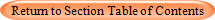Physics and Anesthesiology: Kinetic Theory of GasesIdeal Gas Law

• The ideal gas law can be written PV = nRT where in this case n is the number of moles in this case and also note that PV = NkT where N is the number of atoms in that case.

• Above interactive illustration of PV= NRT is courtesy of Dr. Michael Fowler of the University of Virginia.

• Note the effects on Pressure and Temperature as the Volume is changed as you control piston movement

: